# Chemistry: CBSE Sample Question Paper (2020-21) - 1 Notes | Study Chemistry Class 12 - NEET

## NEET: Chemistry: CBSE Sample Question Paper (2020-21) - 1 Notes | Study Chemistry Class 12 - NEET

The document Chemistry: CBSE Sample Question Paper (2020-21) - 1 Notes | Study Chemistry Class 12 - NEET is a part of the NEET Course Chemistry Class 12.
All you need of NEET at this link: NEET

Class - XII
Chemistry
TIME: 3 Hrs.
M.M: 80

General Instructions:

(a) There are 33 questions in this question paper. All questions are compulsory.
(b) Section A: Q. No. 1 to 16 are objective type questions. Q. No. 1 and 2 are passage based questions carrying 4 marks each while Q. No. 3 to 16 carry 1 mark each. MCQs or Reason Assertion Type based on given passage each carrying 1 mark.
(c) Section B: Q. No. 17 to 25 are Short Answer Questions and carry 2 marks each.
(d) Section C: Q. No. 26 to 30 are Short Answer Questions and carry 3 marks each.
(e) Section D: Q. No. 31 to 33 are Long Answer Questions carrying 5 marks each.
(f) There is no overall choice. However, internal choices have been provided.
(g) Use of calculators and log tables is not permitted.

Section - A

Objective Type

1. Read the passage given below and answer the following questions : (1 x 4 = 4)
Q.1. The potential difference between the two electrodes of a galvanic cell is called the cell potential and is measured in volts. The cell potential is the difference between the electrode potentials (reduction potentials) of the cathode and anode. It is called the cell electromotive force (emf) of the cell when no current is drawn through the cell. It is now an accepted convention that we keep the anode on the left and the cathode on the right while representing the galvanic cell. A galvanic cell is generally represented by putting a vertical line between metal and electrolyte solution and putting a double vertical line between the two electrolytes connected by a salt bridge.
In a galvanic cell, the following cell reaction occurs:
Zn(s) + 2Ag+(aq) → Zn2+(aq) + 2Ag(s)
E°cell = +1.56 V
The following questions are multiple choice questions. Choose the most appropriate answer:

(i) What is the direction of the flow of electrons?
(a) First from silver to zinc, then the direction reverses
(b) Silver to zinc
(c) First from zinc to silver, then the direction reverses
(d) Zinc to silver
Ans. d
Solution. Zinc to silver is the flow of electrons.

(ii) How will concentration of Zn2+ ions and Ag+ ions be affected when the cell functions ?
(a) Concentration of both Zn2+ and Ag+ ions increase
(b) Concentration of Zn2+ increases and Ag+ ions decreases
(c) Concentration of Zn2+ decreases and Ag+ ions increases
(d) Concentration of both Zn2+ and Ag+ ions decreases
Ans. b

(iii) Name the cell which is generally used in inverters?
(a)
Mercury cell
(b) Leclanche cell
(d) Lithium ion battery
Ans. c

OR

Which cell uses a 38% solution of sulphuric acid as an electrolyte ?
(a)
(b) Leclanche cell
(c) Lithium ion battery
(d) Fuel cell
Ans. Lead storage battery is the most common secondary cell. It consists of a lead anode and a grid of lead packed with lead oxide (PbO2) as cathode. A 38% solution of sulphuric acid is used as electrolyte.

(iv) The standard electrode potential for Daniell cell is 1.1V. Calculate the standard Gibbs energy for the reaction : Zn(s) + Cu2+(aq) → Zn2+(aq) + Cu(s).
(a)
215.36 kJ mol–1
(b) –212. 27 kJ mol–1
(c) 212.27 kJ mol–1
(d) –218  kJ mol–1
Ans. b
Solution.

rG° = –nFE°tell
n in the above equation is 2,
F = 96487 C mol–1 and E°tell = 1.1 V
Therefore, ∆rG° = –2 × 1.1V × 96487 C mol–1
r = – 21227 J mol–1
= – 212.27 kJ mol–1

Q.2. Products of electrolysis depend on the nature of material being electrolysed and the type of electrodes being used. If the electrode is inert (e.g., platinum or gold), it does not participate in the chemical reaction and acts only as source or sink for electrons. On the other hand, if the electrode is reactive, it participates in the electrode reaction. Thus, the products of electrolysis may be different for reactive and inert electrodes. Aqueous copper sulphate solution and aqueous silver nitrate solution are electrolysed by 1 ampere current for 10 minutes in separate electrolytic cells.
In these questions, a statement of assertion followed by a statement of reason is given. Choose the correct answer out of the following choices.
(a) Assertion and reason both are correct statements and reason is correct explanation for assertion.
(b) Assertion and reason both are correct statements but reason is not correct explanation for assertion.
(c) Assertion is correct statement but reason is wrong statement.
(d) Assertion is wrong statement but reason is correct statement.
(i) Assertion : The mass of copper and silver, deposited on the cathode be same.

Reason : Copper and silver have different equivalent masses.
Ans. d
Solution.

W = itE/96500
= 1 × 10 × 60 × 31.8/96500 for copper.
It will be different for silver since the equivalent weight of silver is different.

(ii) Assertion : At equilibrium condition Ecell = 0 or ΔrG = 0.
Reason : Ecell is zero when both electrodes of the cell are of the same metal.
Ans. b

(iii) Assertion : The negative sign in the expression EZn2+/Zn = – 0.76V means Zn2+ cannot be oxidised to Zn.
Reason :
Ans. It shows that the reduced form of (Zn) is not stable. It is difficult to reduce Zn2+ to Zn. Rather the reverse reaction i.e Zn can get oxidised to Zn2+ and Hwill get reduced as it is stabler among both the reduced species.

(iv) Assertion : In a galvanic cell, chemical energy is converted into electrical energy.
Reason : Redox reactions provide the chemical energy to the cell.
Ans. a
Solution. The redox reactions provide the chemical energy to the galvanic cell which is converted into electrical energy.

OR
Assertion : Copper sulphate cannot be stored in zinc vessel.
Reason : Zinc is less reactive than copper.
Ans. c
Solution. Copper sulphate cannot be stored in zinc vessel as zinc is more reactive than copper.

Following questions (No. 3 -11) are Multiple Choice Questions carrying 1 mark each: Q3. Compounds A and C in the following reaction are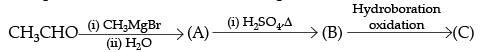(a) identical
(b) positional isomers
(c) functional isomers
(d) optical isomers
Ans. b
Solution.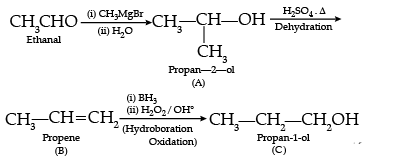OR
Dinucleotide is obtained by joining two nucleotides together by phosphodiester linkage. Between which carbon atoms of pentose sugars of nucleotides are these linkages present?
(a) 5' and 3'
(b) 1' and 5'
(c) 5' and 5'
(d) 3' and 3'
Ans. a
Solution. These linkages are present between 5' and 3' of pentose sugars of nucleotides.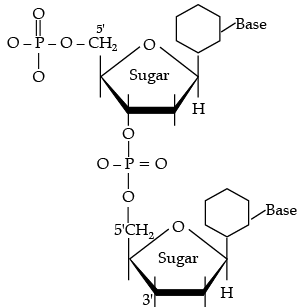Q4. Which of the statements about solutions of electrolytes is not correct ?
(a) Conductivity of solution depends upon size of ions.
(b) Conductivity depends upon viscosity of solution.
(c) Conductivity does not depend upon solvation of ions present in solution.(d) Conductivity of solution increases with temperature.
Ans. b
Solution.

NaCl → Na+ + Cl-
H2O → H+ OH-
Na+ + e- → Na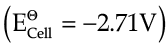H+ + e- → 1/2H2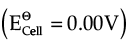At cathode,

H2O + e-→ 1/2H2 + OH-     
At anode, two reactions are possible.

Cl- → 1/2Cl2 + e-;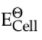= 1.36 V

2H2O → O2 + 4H+ + 4e-;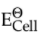= 1.23V    

Q.5. When 1 mol CrCl3⋅6H2O is treated with excess of AgNO3, 3 mol of AgCl are obtained. The formula of the complex is:
(a)
[CrCl3(H2O)3]⋅3H2O.
(b) [CrCl2(H2O)4]Cl⋅2H2O.
(c) [CrCl(H2O)5]Cl2⋅H2O.
(d) [Cr(H2O)6]Cl3.
Ans. d
Solution. [Cr(H2O)6]Cl3 will be formed as we are getting 3 moles of AgCl which shows that the chloride ions are not bound to the complex, as bound ligands cannot separate and form precipitates.

Q.6. Which is the correct increasing order of boiling points of the following compounds ? 1-Bromoethane, 1-Bromopropane, 1-Bromobutane, Bromobenzene
(a) Bromobenzene < 1-Bromobutane  < 1-Bromopropane < 1-Bromoethane
(b) Bromobenzene < 1-Bromoethane  < 1-Bromopropane < 1-Bromobutane
(c) 1-Bromopropane < 1-Bromobutane  < 1-Bromoethane < Bromobenzene
(d) 1-Bromoethane < 1-Bromopropane  < 1-Bromobutane < Bromobenzene
Ans. d
Solution. Boiling point increases with increase in molecular mass of the alkyl halide.

Q.7. Which of the following compounds will give butanone on oxidation with alkaline KMnO4 solution ?
(a)
Butan-1-ol
(b) Butan-2-ol
(c) Both of these
(d) None of these
Ans. a
Solution. Potassium phthalimide is the source of nitrogen in Gabriel synthesis.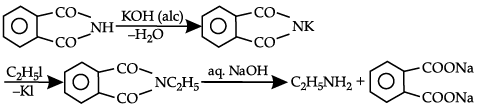OR
Which of the following reagents would not be a good choice for reducing an aryl nitro compound to an amine ?
(a) H2(excess)/Pt
(b) LiAlH4 in ether
(c) Fe and HCl
(d) Sn and HCl
Ans. b
Solution.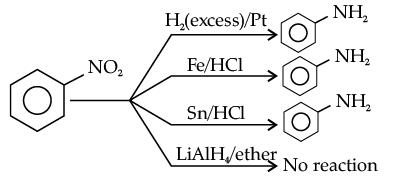Q.8. Which of the following is not true about the ionic solids ?
(a)
Bigger ions form the close-packed structure.
(b) Smaller ions occupy either the tetrahedral or the octahedral voids depending upon their size.
(c) Occupation of all the voids is not necessary.
(d) The fraction of octahedral or tetrahedral voids occupied depends upon the radii of the ions occupying the voids.
Ans. d
Solution. In ccp, unit cell has 8 tetrahedral voids.

OR
In the cubic close packing, the unit cell has __________.
(a)
4 tetrahedral voids each of which is shared by four adjacent unit cells
(b) 4 tetrahedral voids within the unit cell
(c) 8 tetrahedral voids each of the which is shared by four adjacent unit cells
(d) 8 tetrahedral voids within the unit cells
Ans. d
Solution. In ionic solids, cations occupy the voids, and this depends on the stoichiometry of the compounds not on the radius of the ions.

Q.9. Hinsberg's reagent which is used to test amines is
(a)
Benzene sulphonamide
(b) Benzenediazonium chloride
(c) Benzenesulphonyl chloride
(d) Acetanilide
Ans. d
Solution. Heisenbuergs reagent is benzene sulphonyl chloride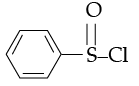2 phenyl prop amide

It can example of mothman Bromamide reaction.

Q.10. Which of the following is most effective in coagulating positively charged methylene blue sol?
(a)
Na3PO4
(b) K4[Fe(CN)6]
(c) Na2SO4
(d) NaNO3
Ans. b
Solution. K4[Fe(CN)6]/[Fe(CN)6]4–.

Q.11. The CFSE for octahedral [CoCl6]4– is 18,000 cm–1. The CFSE for tetrahedral [CoCl4]2– will be
(a)
18,000 cm–1
(b) 16,000 cm–1
(c) 8,000 cm–1
(d) 20,000 cm–1
Ans. c
Solution. In starch, glucose monomers are in α- configuration, while in cellulose, glucose monomers are in β-configuration.

OR
Which of the following options are correct for [Fe(CN)6]3– complex ?
(a) d2sp3 hybridisation
(b) sp3d2 hybridisation
(c) Paramagnetic
(d) Diamagnetic
Ans. b
Solution. Structure of glycogen is similar to amylopectin. It is a branched chain polymer of α D glucose units in which chain is formed by  C1-C4 glycosidic linkage whereas branching occurs by the formation of C1-C6 glycosidic linkage.

In the following questions (Q. No. 12 - 16) a statement of assertion followed by a statement of reason is given. Choose the correct answer out of the following choices.
(a) Assertion and reason both are correct statements and reason is correct explanation for assertion.
(b) Assertion and reason both are correct statements but reason is not correct explanation for assertion.
(c) Assertion is correct statement but reason is wrong statement.
(d) Assertion is wrong statement but reason is correct statement.

Q.12. Assertion (A) : [Fe(CN)6]3– ion shows magnetic moment corresponding to two unpaired electrons.
Reason (R) : Because it has d2sp3 type hybridisation.
Ans. a
Solution. [Fe(CN)6]3– ion has only one unpaired electron and has a d2sp3 hybridisation but it does not explains its weak paramagnetic behaviour.

OR
Assertion (A) : [Cr(H2O)6]Cl2 and [Fe(H2O)6]Cl2 are reducing in nature.
Reason (R) : Unpaired electrons are present in their d-orbitals.
Ans. b
Solution. In the complexes, Co exists as Co2+ and Fe as Fe2+. Both of the complexes become stable by oxidation of metal ion to Co3+ and Fe3+.

Q.13. Assertion (A) : HI cannot be prepared by the reaction of KI with concentrated H2SO4.
Reason (R) : HI has lowest H–X bond strength among halogen acids.
Ans. b
Solution. Both statements are correct but are independent of each other.  HI cannot be prepared by the reaction of KI with concentrated H2SO4 as it results in the formation of HI which further oxidizes to I2 as H2SO4 is a strong oxidizing agent.

Q14. Assertion (A) : SF6 cannot be hydrolysed but SF4 can be.
Reason (R) : Six F atoms in SF6 prevent the attack of H2O on sulphur atom of SF6.
Ans. a
Solution. SF6 is sterically protected due to presence of six F atoms around S atom which prevents the attack of H2O on SF6.

Q.15. Assertion (A) : Presence of a nitro group at ortho or para position increases the reactivity of haloarenes towards nucleophilic substitution.
Reason (R) : Nitro group, being an electron withdrawing group decreases the electron density over the benzene ring.
Ans. a
Solution. Nitro group being electron withdrawing group, decreases the electron density of ring hence increases the reactivity of haloarenes towards nucleophilic substitution.

Q.16. Assertion (A) : Acylation of amines gives a monosubstituted product whereas alkylation of amines gives polysubstituted product.
(Reason) : Acyl group sterically hinders the approach of further acyl groups.
Ans. c
Solution. In alkylation, an amine can react with alkyl halide to form next higher class of amine caused by the presence of electron pair on nitrogen which makes amine to behave as nucleophile and alkyl halide thus undergo nucleophilic substitution reaction. When primary and secondary amines react with acid chlorides, anhydrides and esters to give monosubstituted amides as products. Acylation is carried out in the presence of a base stronger than the amine like pyridine which causes the shift of the equilibrium to the right side.

OR
Assertion (A) : Acetanilide is less basic than aniline.
Reason (R) : Acetylation of aniline results in decrease of electron density on nitrogen.
Ans. a
Solution. Acetanilide is less basic than aniline as in amides the carbonyl group (C = O) is a stronger dipole than N-C dipole. Therefore, the ability of N-C group to act as H-bond acceptor (as a base) is restricted in the presence of a C = O dipole.

Section - B

The following questions (Q.No 17 – 25) are Short Answer Type- I and carry 2 marks each.
Q.17. A 1.00 molar aqueous solution of trichloroacetic acid (CCl3COOH) is heated to its boiling point. The solution has the boiling point of 100.18°C. Determine the van’t Hoff factor for trichloroacetic acid. (Kb for water = 0.512 K kg mol–1).
Ans.

Tb - 100.18°C = 373.18 K
b - 100°C = 373K
ΔTb = iKbm
(373.18 - 373) K = i X 0.512 K kg mol-1 x 1 m
O.18K = i X 0.512 K kg mol-1 X 1m,
i = 0.35

Q.18. Calculate the emf of the following cell at 298 K
Cr(s)|Cr3+ (0.1M)||Fe2+ (0.01M)|Fe(s)
[Given : E°cell = + 0.30 V]
Ans. 2Cr(s) + 3 Fe2+(aq.) → 3Fe(s) + 2Cr3+(aq.) n = 6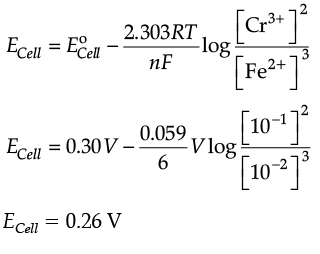Q.19. Although phenoxide ion has more number of resonating structures than carboxylate ion, carboxylic acid is a stronger acid than phenol. Give two reasons.
Ans.(i) Phenoxide ion has non-equivalent resonance structures in which the negative charge is at the lesser electronegative carbon atom whereas in case of carboxylate ion both the resonating structures are equivalent.
(ii) The negative charge is delocalised over two electronegative oxygen atoms in carboxylate ion whereas in  phenoxide ion, the negative charge less effectively delocalises over one oxygen atom and less electronegative carbon atoms. So, the carboxylate ion is more resonance stabilised than phenoxide ion. Thus, the release of proton from carboxylic acid is much easier than from phenol. Hence, carboxylic acid is a stronger acid than phenol.

Q.20. An element with density 2.8 g cm–3 forms a fcc unit cell with edge length 4 × 10–8 cm. Calculate the molar mass of the element. (Given: NA = 6.022 × 1023 mol–1)
Ans.
d = 2.8 g cm–3; z = 4 (for fcc), a = 4 × 10–8 cm;
NA = 6.022 × 1023 mol–1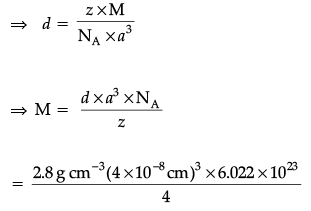⇒ M = 2.8 × 16 × 10–1 × 6.022
= 26.98 g mol–1

Q.21. Write the equations for the preparation of 1-bromobutane from:
(i) 1-butanol
(ii) but-1-ene
Ans.
(i)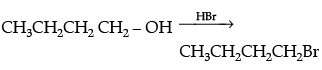(ii)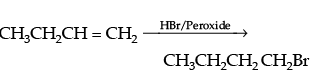OR
Although chlorine is an electron withdrawing group, yet it is ortho-1 para-directing in electrophilic aromatic substitution reactions. Explain why it is so?
Ans. Although chlorine is an electron withdrawing group, yet it is ortho-para directing in nature in electrophilic aromatic substitution because when chlorine is present in benzene ring, it releases electron by resonance whereas it acts as withdrawing group only through inductive effect. By inductive effect, chlorine atom destabilizes the intermediate carbocation formation but by resonance, chlorine atom stabilises the intermediate carbocation and effect is more at o-and p-positions. Resonance effect opposes inductive effect. Inductive effect is stronger than resonance effect due to which reactivity is controlled by inductive effect and orientation by resonance.

Q.22. What is crystal field splitting energy? Which character of coordination compounds is determined by it? What will be the correct sequence of absorption of wavelength of light in the visible region for the complexes.
[Co(NH3)6]3+, [Co(CN)6]3−, [Co(H2O)6]3+
Ans. (i) Crystal field splitting energy is the difference in magnitude in energy between the two sets of d orbitals. i.e. t2g and eg. The colour of the coordination compounds is determined by it.
(ii) The correct order of absorption of wavelength of light in the visible region will be [Co(H2O)6]3+ > [Co(NH3)6]3+ > [Co(CN)6]3–
Since as crystal field splitting depends on the strength of the ligand. Stronger the ligand, greater is the crystal field splitting, greater the energy absorbed, smaller is the wavelength absorbed.

OR
Define crystal field splitting energy. On the basis of crystal field theory, write the electronic configuration for d4 ion if Δo < P.
Ans. It is the magnitude of difference in energy between the two sets of d orbital i.e. t2g and eg
t32ge1g

Q.23. Explain redox potential.
Reduction potentials of some ions are given below. Arrange them in decreasing order of oxidising power.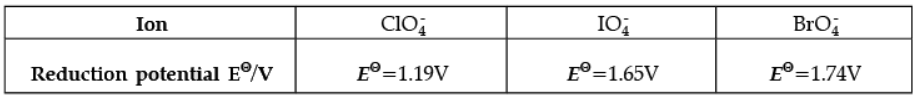Ans. Redox potential (also known as reduction / oxidation potential) is a measure of the tendency of a chemical species to acquire electrons from or lose electrons to an electrode and thereby be reduced or oxidised respectively. Redox potential is measured in volts (V), or millivolts (mV). The more positive the reduction potential of a species, the greater the species' affinity for electrons and tendency to be reduced.
The higher the reduction potential, the higher is its tendency to get reduced. Hence, the order of oxidising power is: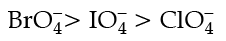Q.24 Write the chemical equations involved in the following reactions:
(i)
(ii) Carbylamine reaction.
Ans.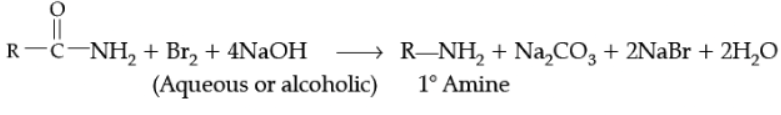Example,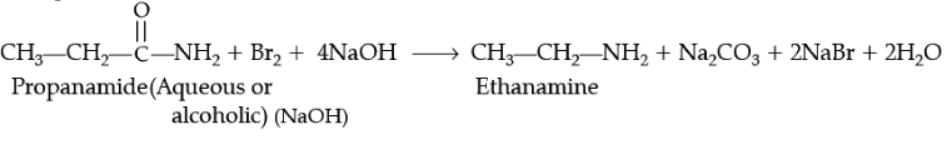Q.25. Give reasons for the following:
(i) Transition metals have high enthalpies of atomization.
(ii) Manganese has lower melting point even though it has a higher number of unpaired electrons for bonding.
Ans.

(i) Because of strong interatomic interactions / Strong metallic bonding between atoms.(ii) Due to stable 3d5 configuration , interatomic interaction is poor between unpaired electrons.
Detailed Answer: (i) The transition elements have high enthalpies of atomization because they have large number of unpaired electrons in their atoms. This results in stronger interatomic interaction and stronger bonding between atoms.
(ii) Mn has low melting point because of 3d54s2 configuration, which is highly stable and delocalised, so are not available for bonding, as a result interatomic forces becomes weaker. Hence Mn has low melting point bounded half-filled a orbital electrons with nucleus results in strong interatomic interaction.

Section - C

Q.No 26 -30 are Short Answer Type - II carrying 3 marks each.
Q26. (i) Account for the following:
(a)
Transition metals show variable oxidation states.
(b) Zn, Cd and Hg are soft metals.
(c) E° value for the Mn3+/Mn2+ couple is highly positive (+1.57 V) as compared to Cr3+/Cr2+.
Ans. (i) (a) The valence electrons of transition metals are in (n-1)d and ns orbitals. As there is almost little energy difference between these orbitals, both the energy levels can be used for bond formation. Thus, they exhibit variable oxidation states.
(b) Because they contain fully filled d-orbitals, no unpaired d electrons are present resulting in weak metallic bonding.

(ii) Account for the following
(a) The highest oxidation state is exhibited in oxoanions of transition metals.
(b) HCl is not used to acidify KMnO4 solution.
(c) Transition metals have high enthalpy of atomisation.
Ans. (ii) (a) Due to ability of oxygen to form multiple bonds to metal.
(b) HCl is oxidized to chlorine.
(c) Due to strong interatomic bonding.

(ii) (a) Due to high electronegativity and small size, oxygen acts as a strong oxidising agent. This results in oxygen’s ability to oxidise the metal to attain highest oxidation state.
(b) As KMnO4 is a very strong oxidising agent, it oxidizes HCl resulting in evolution of chlorine gas. Therefore, HCl is not used to acidify KMnO4 solution.

Q.27. (i) Of the two hydroxy organic compounds ROH and R′OH, the first one is basic and other is acidic in behaviour. How is R different from R′ ?
(ii) Arrange the following compound groups in the increasing order of their property indicated:

(a) p -nitrophenol, ethanol, phenol (acidic character)
(b) P ropanol, Propane, Propanal (boiling point)
Ans. (i) R is aliphatic while R′ is aromatic.
(ii) (a) ethanol<phenol<p-nitrophenol
(b) propane <propanal<propanol

Q.28. The following curve is obtained when molar conductivity (Lm) is plotted against the square root of concentration, c½ for two electrolytes A and B: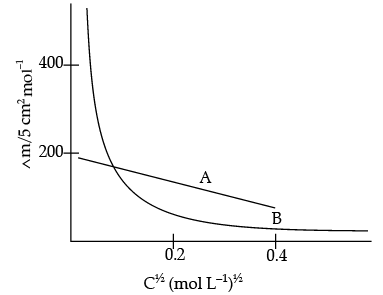(i) How do you account for the increase in the molar conductivity of the electrolyte A on dilution ?
(ii) As seen from the graph, the value of limiting molar conductivity (∧°m) for electrolyte B cannot be obtained graphically. How can this value be obtained ?
(iii) Define limiting molar conductivity.
Ans. (i) As seen from the graph, electrolyte A is a strong electrolyte which is completely ionised in solution. With dilution, the ions are far apart from each other and hence the molar conductivity increases.
(ii) To determine the value of limiting molar conductivity for electrolyte B, indirect method based upon Kohlrausch law of independent migration of ions is used.
(iii) When concentration approaches zero, the molar conductivity is known as limiting molar conductivity.

Q.29. (i) What happens when CH3 – O – CHis heated with HI ?
(ii) Explain mechanism for acid catalysed hydration of ethene: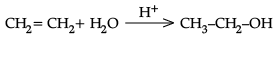Ans.

(i) CH- O - CH3 + HI → CH3 - OH + CH3 - I
(ii) Protonation of alkene to form carbocation by electrophilic attack of H3O+.

H2O + H→ H3O+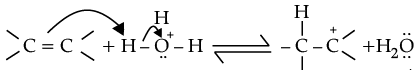Nucleophilic attack of water on carbocation.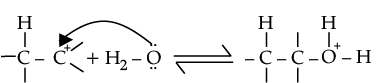Deprotonation to form an alcohol.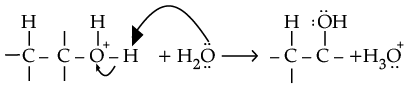OR
(i) Why pKa of F – CH2 – COOH is lower than that of Cl – CH2 – COOH ?
(ii) Write the product in the following reaction
CH3 – CH = CH – CH2CN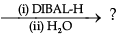(iii) How can you distinguish between propanal and propanone ?
Ans.
(i) In FCH2 – COOH, fluorine is more electron withdrawing and has stronger -I effect than chlorine in ClCH2 – COOH. So, FCH2COOH is more acidic than ClCH2COOH hence its pKa value is lesser than ClCH2COOH.
(ii) CH3 – CH = CH – CH2CN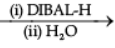CH3 – CH = CH – CH2 – CHO
Pent-3-ene-1-al

(iii) Propanal and propanone can be differentiated by Tollen’s reagent i.e. propanal will give silver mirror but propanone will not.
CH3 – CH2 – CHO + 2[Ag(NH3)2]+
CH3 – CH2 – COO + 2Ag¯+ H2O + 4NH3
(Silver mirror)
Q.30. (i) Write the mechanism of the following reaction: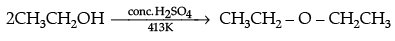(ii) Write the equations involved in the acetylation of Salicylic acid.
Ans.
(i)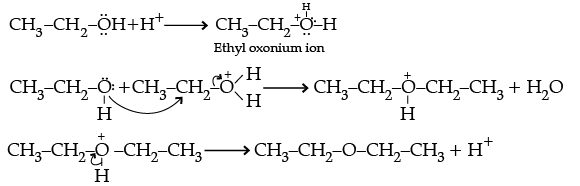(ii)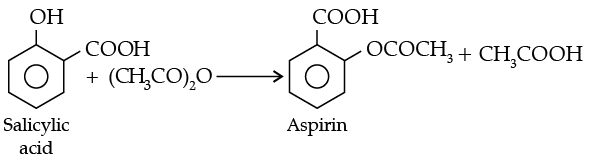Section - D

Q.No 31 to 33 are Long Answer Type carrying 5 marks each.
Q.31. (i) Write the IUPAC name of the ionisation isomer of the coordination compound [Co(NH3)5Br]SO4. Give one chemical test to distinguish between the two compounds.
(ii) How is a double salt different from a complex ?
(iii) What is the denticity of coordination compound used for the treatment of lead poisoning ?
(iv) Which of the following is more stable complex and why ?
[Co(NH3)6]3+ and [Co(en)3]3+.
Ans. (i) The ionisation isomer is [Co(NH3)5SO4]Br. The IUPAC name is Pentaamminesulphatocobalt (III) bromide.
The isomer [Co(NH3)5Br]SO4 gives a white precipitate of BaSO4 with BaCl2 solution whereas the isomer [Co(NH3)5SO4]Br does not form this precipitate. (or any other relevant test)
(ii)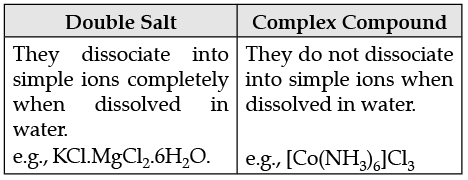(iii) In lead poisoning, EDTA (Ethylene diamine tetraacetate) is used as ligand which is hexadentate i.e., the denticity of EDTA is 6. EDTA binds with metal in octahedral manner by two N-atoms and four acetate oxygen atoms.
(iv) [Co(en)3]3+: Because (en) is a chelating ligand/ bidentate ligand.

Q.32. (i) State a condition under which a bimolecular reaction is kinetically first order reaction.
(ii) With the help of a diagram, explain the physical significance of energy of activation (Ea) in chemical reactions.

OR

All energetically effective collisions do not result in a chemical change. Explain with the help of an example.
(i)
(a) Although both [NiCl4]2– and [Ni(CO)4] have sp3 hybridisation yet [NiCl4]2– is paramagnetic and [Ni(CO)4] is diamagnetic. Give reason. (Atomic no. of Ni = 28)
(b) Write the electronic configuration of d5 on the basis of crystal field theory when:
(i) Δo< P and (ii) Δo> P
(ii) (a) F2 has lower bond dissociation enthalpy than Cl2. Why ?
(b) Which noble gas is used in filling balloons for meteorological observations ?
(c) Complete the following equation:
XeF2 + PF5
Ans. (i) Let us take a bimolecular reaction:
A + B Product
Rate = k [A] [B]
When concentration of [B] is taken in excess then rate law will become:
Rate = k [A]
where, k = constant

The rate depends only on one of the reactant as there is negligible change in its concentration so it is bimolecular but is of first order.
(ii) The excess energy which must be supplied to the reactants to undergo chemical reactions is called activation energy Ea. It is equal to the difference between the threshold energy ET, needed for the reaction and the average of all the reacting molecules, ER.

Activation energy = Threshold energy – Average kinetic energy of the reacting molecule.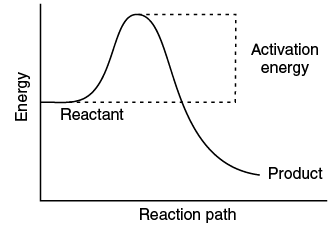OR
Effective collision leads to the formation of products implying that during collisions molecules collide with sufficient kinetic energy called threshold energy (Threshold Energy = Activation energy + Energy possessed by reacting species) and proper orientation leads to a chemical change because it increases the  old bond breaking between reactant molecules and formation of the new ones in products. For example : Formation of methanol from bromoethane depends upon the orientation of the reactant molecules. Thus, proper orientation of reactant molecules leads to bond formation while improper orientation does not allow bond formation and hence no products are formed. To estimate effective collisions, another factor P which is called as probability or steric factor is introduced.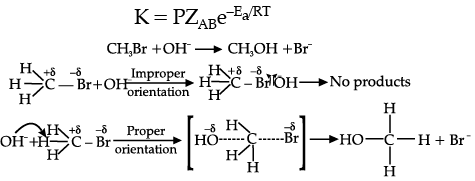(i) (a) In [NiCl4]2–, Cl is a weak field ligand due to which there are two unpaired electrons in 3d orbital whereas in [Ni(CN)4]2–, CN is a strong field ligand due to which pairing leads to no unpaired electron in 3d- orbital/ or structural representation.
(b) (i) t2g3eg(ii) t2g5eg0

(i) In [NiCl4]2– Ni is in +2 oxidation state and each Cl– donates a pair of electron. So, Cl acts as a weak ligand and does  not cause any forced pairing. Thus, electrons remains unpaired making it paramagnetic. In [Ni(CO)4], Ni is in zero oxidation state and CO acts as a strong ligand causing forced pairing. Thus, no electron remains unpaired making it diamagnetic.
(ii) (a) F2 has lower bond dissociation energy than Cl2 because the size of F2 is much smaller than Cl2 as a result interelectronic repulsion works and make F2 weak.
(b) Helium is used in filling balloons for meteorological observations.
(c) XeF2 + PF5 → [XeF]+ [PF6]

Q.33. (i) For a reaction A + B → P, the rate is given by
Rate = k[A] [B]2
(a)
How is the rate of reaction affected if the concentration of B is doubled ?
(b) What is the overall order of reaction if A is present in large excess ?
(ii) A first order reaction takes 30 minutes for 50% completion. Calculate the time required for 90% completion of this reaction. (log 2 = 0.3010)

OR
Explain the following:
(i) Same substance can act both as colloids and crystalloids.
(ii) Artificial rain is caused by spraying salt over clouds.
Ans. (i) A + B → P
Rate = k [A ] [B]2
(a) When concentration of B is doubled it means concentration of B becomes 2 times.

Thus, Rate = k[A]1 [2B]2
= k[A ] [4B2]
So, the rate becomes 4 times.
(b) Order of reaction is the number of molecules whose concentration alters after the reaction. If A is present in excess i.e., its concentration is unaffected.

So, rate depends only on the concentration of B. as k = [B]2
Thus, the reaction is of second order.
(ii) For the 1st order reaction: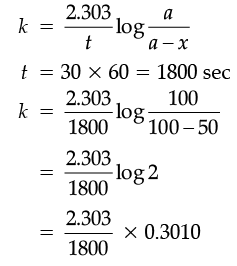And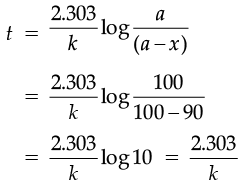By putting the value of k here, we get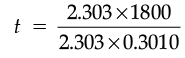OR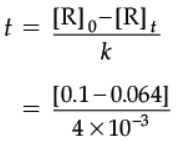The equation for the zero order reaction is:
[A]=[A0] – kt
Given:
[A0] = 0.1 M

[A] = 0.064

K = 4 × 10–3 M/s
Putting the values in the equation, and solving the equation for t, we get:
0.064 = 0.1 – (4 × 10–3 × t)
0.064 – 0.1 = – (4 × 10–3 × t)
– 0.036 = – (4 × 10–3 × t)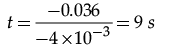OR
(i) The nature of the substance whether colloid or crystalloid depends upon size of the solute particles. When the size of solute particles lies between 1 to 1000 nm, it behaves as a colloid.
(ii) The colloidal water particles of the clouds get neutralized and coagulated to bigger water drops by spraying salt over clouds and as a result artificial rain is caused.

The document Chemistry: CBSE Sample Question Paper (2020-21) - 1 Notes | Study Chemistry Class 12 - NEET is a part of the NEET Course Chemistry Class 12.
All you need of NEET at this link: NEETUse Code STAYHOME200 and get INR 200 additional OFF

## Chemistry Class 12

110 videos|306 docs|259 tests

### How to Prepare for NEET

Read our guide to prepare for NEET which is created by Toppers & the best Teachers

Track your progress, build streaks, highlight & save important lessons and more!

,

,

,

,

,

,

,

,

,

,

,

,

,

,

,

,

,

,

,

,

,

;